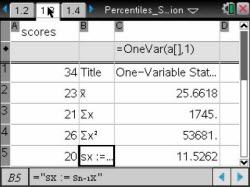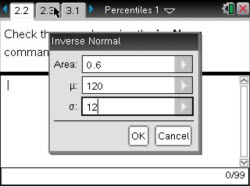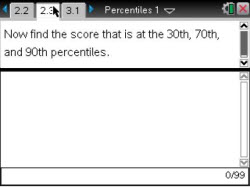### Mathematics lessons for IB® Diploma Programme

Analysis and Approaches | Probability and Statistics

## Percentiles

### Activity Overview

Students use the area to the left of a value in a normal distribution to find its percentile and then reverse the process to find the value for a given percentile.

## Key Steps## Step 1

In Problem 1, students find the mean, standard deviation, and normal distribution in order to find the percentile of a given score. They will also use the Normal Cdf command.## Step 2

Problem 2 asks students to find the score given the percentile. Students reverse the method used in problem 1. This reversal is done by using a command called Inverse Normal.## Step 3

In problem 3, students find the solutions to each scenario given. These scenarios include finding the higher percentile given two scores from different distributions, estimating a score, and finding a possible score.